﻿ 中医脉诊信号的无监督聚类分析研究
«上一篇文章快速检索 高级检索

 智能系统学报2018, Vol. 13Issue (4): 564-570  DOI: 10.11992/tis.2017030300

### 引用本文FENG Bing, LI Shaozi. Unsupervised clustering analysis of human-pulse signal in traditional Chinese medicine[J]. CAAI Transactions on Intelligent Systems, 2018, 13(4): 564-570. DOI: 10.11992/tis.201703030.### 文章历史

Unsupervised clustering analysis of human-pulse signal in traditional Chinese medicine
FENG Bing, LI ShaoziSchool of Information Science and Engineering, Xiamen University, Xiamen 361000, China
Abstract: With the development of a more objective basis for traditional Chinese medicine (TCM), objectivity and instrumentation are growing trends in pulse-taking techniques. However, choosing an objective method for interpreting the data collected by newly developed TCM diagnostic machines is a recurring issue in the move toward objective pulse-taking diagnosis. Traditional machine learning methods rely heavily on annotated pulse-diagnosis data; however, in TCM practice, different doctors make different annotations based on their different experiences in pulse manifestation. After comparing various feature extraction methods and clustering schemes, in this paper, we propose an improved unsupervised human-pulse identification approach. In this method, we use the dual-tree complex wavelet transform (DTCWT) to preprocess data and Mel-frequency cepstral coefficients (MFCCs) to extract features. Before the data are annotated by TCM experts, we applied the fuzzy c-means (FCM) clustering algorithm to the signal features to classify thick lines, after which further detailed classifications can be made. The experimental results show that excellent classification effects can be obtained by this method, which provides an objective basis for TCM pulse diagnosis.
Key words: pulse diagnosis    machine learning    unsupervised learning    clustering analysis    DTCWT    TCM objectification    MFCC    FCM

1 相关研究工作

2 中医脉诊的无监督分类方法

2.1 基于双树复小波变换的去除基线漂移方法

 $\psi (t) = {\psi _h}(t) + j{\psi _g}(t)$ (1)

 $\begin{array}{c}\psi \left( {x,y} \right) = \psi \left( x \right)\psi \left( y \right) = \left[ {{\psi _h}\left( x \right) + j{\psi _g}\left( x \right)} \right]\left[ {{\psi _h}\left( y \right) + j{\psi _g}\left( y \right)} \right] = {\psi _h}\left( x \right){\psi _h}\left( y \right) - {\psi _g}\left( x \right){\psi _g}\left( y \right) + j\left[ {{\psi _g}\left( x \right){\psi _h}\left( y \right) + {\psi _h}\left( x \right){\psi _g}\left( y \right)} \right]\end{array}$ (2)

 $\psi_h (x){\psi _h}(y) - {\psi _g}(x) {\psi _g}(y)$ (3)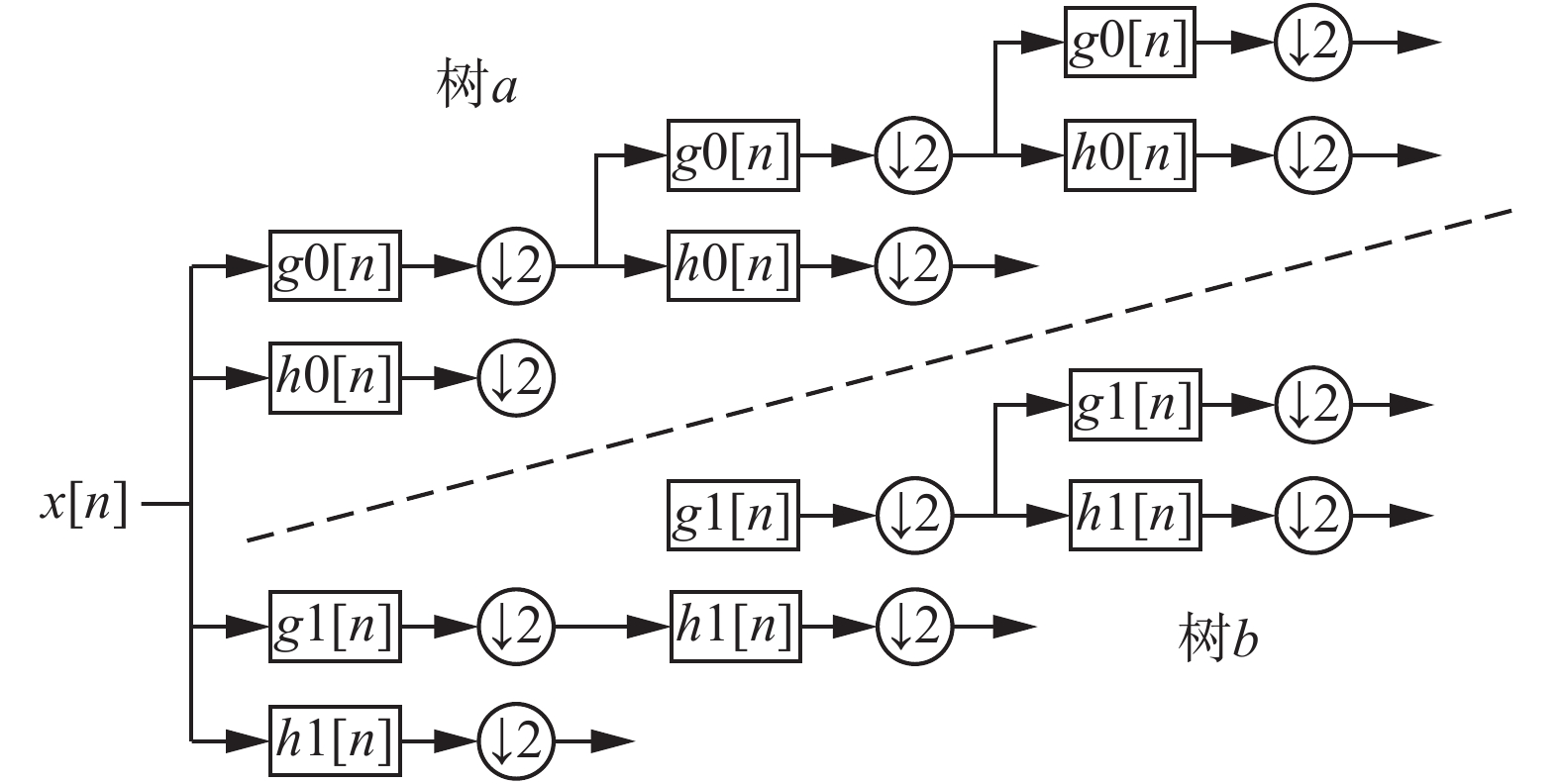Download: 图 1 双树复小波二叉树结构 Fig. 1 Double tree complex wavelet binary tree structure

2.2 基于小波变换的脉象信号的去噪与归一化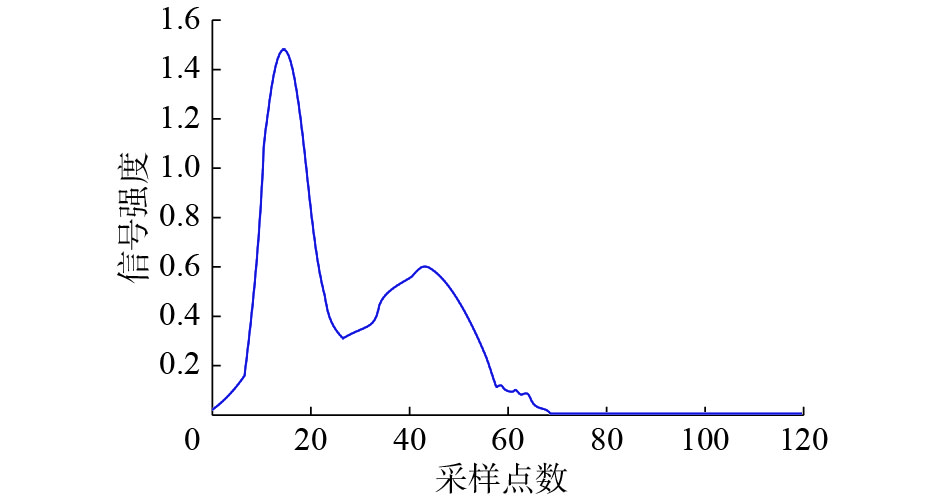Download: 图 2 脉搏信号归一化单心动周期 Fig. 2 Pulse signal normalized single cardiac cycle
2.3 基于梅尔倒谱系数的特征提取

 ${X}[k]={H}[k]{E}[k]$ (4)

 $\log||{X}[k]||=\log||{H}[k]||+\log||{E}[k]||$ (5)

 $x[k]=h[k]+e[k]$ (6)

MFCC特征的特点，是在频率坐标轴上非均匀的滤波。具体来说：在低频区有很多滤波器，分布较为密集，而在高频区域，滤波器的数目就变得较少，分布也变得稀疏(如图3)。这样的特点，可以把特征的关注点放在某些特定的频率，比较符合脉诊信号在中医诊断中的特点，因此可以较好地抓住相关信号的本质特征。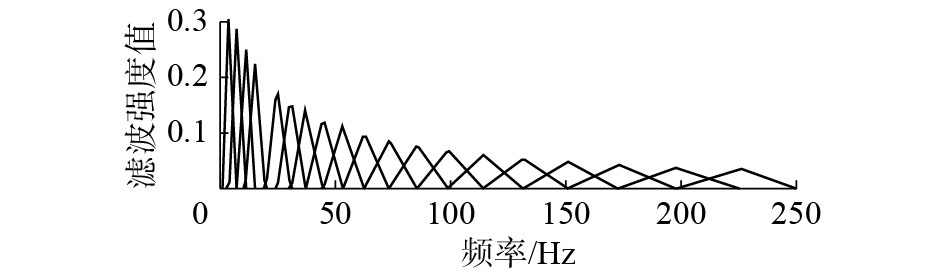Download: 图 3 MFCC非线性频谱(横轴与纵轴分别代表频率与滤波) Fig. 3 MFCC non-linear spectrum (horizontal for frequency, vertical for filter)
2.4 基于Fuzzy C-means (FCM)的聚类Download: 图 4 脉象信号处理流程 Fig. 4 Structure model of group organizations

FCM算法的做法，是首先将n个向量xi(i=1，2， $\cdots$ n)分为c个模糊群，并找出每个群的聚类中心，接下来非相似性指标的计算价值函数，令这些聚类中心能使价值函数达到最小。传统聚类算法(含K-means算法等)，可以被称作是硬聚类算法HCM(hard clustering method)，而FCM算法与HCM这样的硬聚类算法之间的重要区别，是在FCM采用了模糊的划分，从而使得单个给定采样点均使用[0，1]的隶属度数值，来明确其归属单个群的状况。与前面介绍的模糊划分相同，整体分类中的隶属度矩阵U也是由取值在[0，1]的元素构成的。同时，归一化的规则明确了单个数据集的所相加得到的隶属度总和必须等于1。

 $\sum\limits_{i = 1}^c {{u_{ij}}} = 1,\forall j = 1,2, \cdots ,n$ (7)

 $J\left( {{{U}},{c_1}, \cdots ,{c_c}} \right) = \sum\limits_{i = 1}^c {{J_i}} = \sum\limits_{i = 1}^c {\sum\limits_j^n {u_{ij}^md_{ij}^2} }$ (8)

 $\begin{array}{*{20}{c}}\bar J\left( {{{U}},\;{c_1}, \cdots ,{c_c},{\lambda _1}, \cdots ,{\lambda _n}} \right) = \\J\left( {{{U}},\;{c_1}, \cdots ,{c_c}} \right) + \displaystyle\sum\limits_{j = 1}^n {{\lambda _j}\left( {\displaystyle\sum\limits_{i = 1}^c {{u_{ij}} - 1} } \right) = } \\[8pt]{\displaystyle\sum\limits_{i = 1}^c {\sum\limits_j^n {u_{ij}^md_{ij}^2} } + \sum\limits_{j = 1}^n {{\lambda _j}\left( {\sum\limits_{i = 1}^c {{u_{ij}} - 1} } \right)} }\end{array}$ (9)

 ${c_i} = \frac{{\displaystyle\sum\limits_{j = 1}^n {u_{ij}^m{x_j}} }}{{\displaystyle\sum\limits_{j = 1}^n {u_{ij}^m} }},\;\;\;\;{u_{ij}} = \frac{1}{{\displaystyle\sum\limits_{k = 1}^c {{{\left( {\frac{{{d_{ij}}}}{{{d_{kj}}}}} \right)}^{2/\left( {m - 1} \right)}}} }}$ (10)

3 实验结果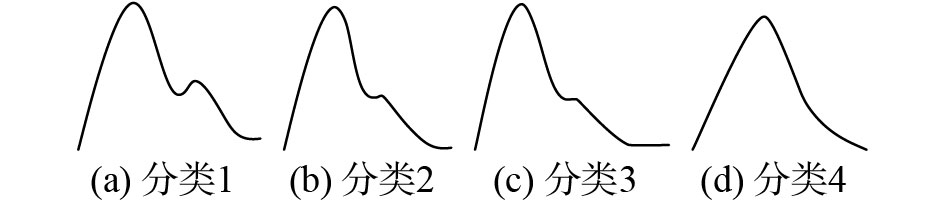Download: 图 5 单脉搏信号粗分类基本类型 Fig. 5 Structure model of group organizations表 1 多聚类方案与特征源实验效果对比 Tab.1 Comparison experiment of multi-clustering scheme and feature source %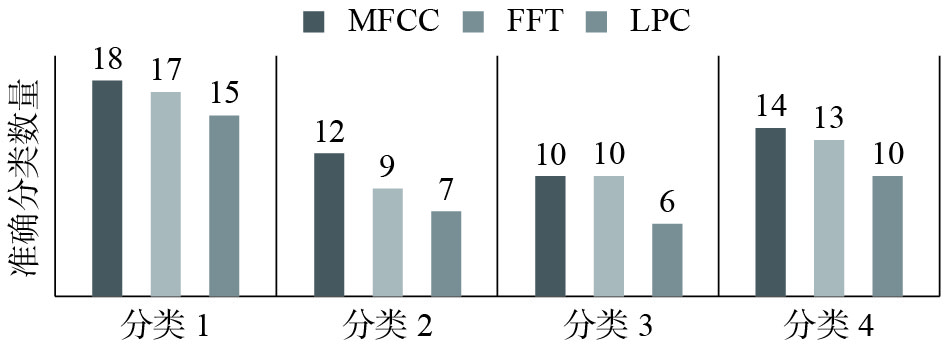Download: 图 6 3种特征方案对单脉搏信号的准确分类数量 Fig. 6 Accurate classification of single pulse signals by three feature schemes
4 结束语

  魏红, 徐刚. 从中医整体、动态、平衡观论脉诊客观化研究[J]. 中医杂志, 2014, 55(1): 25-27. WEI Hong, XU Gang. Research on objectifying pulse examination from overall, dynamic and balanced view of traditional Chinese medicine[J]. Journal of traditional Chinese medicine, 2014, 55(1): 25-27. (0)  刘磊, 吴秋峰, 张宏志, 等. 脉诊客观化研究综述[J]. 智能计算机与应用, 2013, 3(3): 20-24. LIU Lei, WU Qiufeng, ZHANG Hongzhi, et al. The objectification research of pulse diagnosis[J]. Intelligent computer and applications, 2013, 3(3): 20-24. (0)  洪光, 高丹. 中医脉诊客观化发展探析[J]. 国际中医中药杂志, 2016, 38(10): 869-871. HONG Guang, GAO Dan. Introduction and reflection on the current status of objectification of Chinese pulse diagnosis[J]. International journal of traditional Chinese medicine, 2016, 38(10): 869-871. DOI:10.3760/cma.j.issn.1673-4246.2016.10.002 (0)  李凯. 脉搏信号采集系统设计及算法研究[D]. 天津: 天津理工大学, 2014. LI Kai. Design of pulse signal collecting system and research of processing algorithm[D]. Tianjin, China: Tianjin University of Technology, 2014. (0)  彭涛. 脉象信号分析与中医脉象证型识别研究[D]. 苏州: 苏州大学, 2008. PENG Tao. Research on analysis of pulse signal and recognition of the tradition Chinese medicine syndrome[D]. Suzhou, China: Soochow University, 2008. (0)  李娜, 邹小娟, 王忆勤. 支气管哮喘与慢性胃炎患者寸口6部脉图的比较研究[J]. 世界科学技术–中医药现代化, 2015, 17(2): 343-349. LI Na, ZOU Xiaojuan, WANG Yiqin. Comparative study on Cun-Kou six-pulse diagram between chronic gastritis and bronchial asthma[J]. World science and technology-modernization of traditional Chinese medicine, 2015, 17(2): 343-349. (0)  张冬雨. 面向脉诊的脉搏信号与血流信号分类研究[D]. 哈尔滨: 哈尔滨工业大学, 2010. ZHANG Dongyu. Research on classification of pulse signal and blood flow signal for pulse diagnosis[D]. Harbin, China: Harbin Institute of Technology, 2010. (0)  郭红霞, 王炳和, 张丽琼, 等. 基于小波包分析和BP神经网络的中医脉象识别方法[J]. 计算机应用研究, 2006, 23(6): 185-187. GUO Hongxia, WANG Binghe, ZHANG Liqiong, et al. Recognition method of TCM pulse-conditions based on wavelet packet analysis and BP neural networks[J]. Application research of computers, 2006, 23(6): 185-187. (0)  ZHANG Shiru, SUN Qingfu. Human pulse recognition based on wavelet transform and BP network[C]//Proceedings of 2015 IEEE International Conference on Signal Processing, Communications and Computing (ICSPCC). Ningbo, China, 2015: 1–4. (0)  王群, 李敏, 庞枫骞, 等. 一种基于时变自回归模型的抗运动干扰心率提取算法[J]. 航天医学与医学工程, 2016, 29(1): 14-20. WANG Qun, LI Min, PANG Fengqian, et al. A motion-resistant heart rate extracting algorithm based on TVAR model[J]. Space medicine and medical engineering, 2016, 29(1): 14-20. (0)  于璐. 基于虚拟仪器的脉搏信号处理方法研究及应用[D]. 沈阳: 中国医科大学, 2009. YU Lu. Research and application of pulse signal processing methods based on virtual instrument[D]. Shenyang, China: China Medical University, 2009. (0)  王欣. 中医三部脉象信号的采集及其关联性研究[D]. 天津: 天津大学, 2014. WANG Xin. The relevance of Cun-guan-chi pulse based on a signal collection system[D]. Tianjin, China: Tianjin University, 2014. (0)  徐黎明, 宿明良, 张广福, 等. 关脉信号数学分析法对比研究[J]. 中国中医药信息杂志, 2005, 12(7): 16-19. XU Liming, SU Mingliang, ZHANG Guangfu, et al. Compared investigation of mathematical analyses for guan pulse tracings[J]. Chinese journal of information on TCM, 2005, 12(7): 16-19. (0)  焦琪玉. 脉象信号的特征提取与分类识别[D]. 长春: 长春理工大学, 2014. JIAO Qiyu. The pulse signals extraction and recognition[D]. Changchun, China: Changchun University of Science and Technology, 2014. (0)  张蔚波, 齐淑敏, 杜丽. 基于频域分析的脉搏波信号研究[J]. 山东建筑大学学报, 2010, 25(4): 419-422. ZHANG Weibo, QI Shumin, DU Li. Study of pulse wave based on frequency domain analysis[J]. Journal of Shandong jianzhu university, 2010, 25(4): 419-422. (0)  周红标. 融合语音和脉搏的多模态情感识别研究[J]. 微电子学与计算机, 2015, 32(6): 5-9. ZHOU Hongbiao. Research of multimodal emotion recognition based on speech and pulse signal[J]. Microelectronics and computer, 2015, 32(6): 5-9. (0)  朴尚哲, 超木日力格, 于剑. 模糊C均值算法的聚类有效性评价[J]. 模式识别与人工智能, 2015, 28(5): 452-461. PIAO Shangzhe, CHAOMURILIGE, YU Jian. Cluster validity indexes for FCM clustering algorithm[J]. PR and AI, 2015, 28(5): 452-461. (0)  康家银, 纪志成, 龚成龙. 一种核C-均值聚类算法及其应用[J]. 仪器仪表学报, 2010, 31(7): 1657-1663. KANG Jiayin, JI Zhicheng, GONG Chenglong. Kernelized fuzzy C-menas clustering algorithm and its application[J]. Chinese journal of scientific instrument, 2010, 31(7): 1657-1663. (0)